Blog

Type Cast operator and Number System in C

21 September, 2018

Type cast :

• It is a concept of converting one data type values into another data type.
• in ‘c’ programming language, “type castings” are classified into two types. i.e
• Explicit type casting
• when we are converting lower data types value into higher data types. Then it is called. Implicit type casting process
• Implicit type casting is under the control of compiler
• As a programmer, it is not required to consider implicit type casting process.
• When we are converting higher data types values into lower data type then it is called Explicit type casting.
• As a programmer it is mandatary to handle explicit type casting process because data overflow will occur

syntax:

datatype1           variable1=value; Datatype2           variable2; variable2=(Data type2) variable1; Ex:1  int i=45; float f; f=1;             //f=(float)i; Ex:-2           double d=123456.789; long intl; l=(long int)d;
• Void main()

{ float f; f=7.6; if(f==7.6)                       // if(float==double)4B==8B by default printf(“welcome”); else printf(“Hello”); o/p: Hello.
• By default , any type of real values is double that why, when we are comparing the float data with double then automatically condition became false.

void main()

{ float f; f=3.8; if(f==3.8f)  ||  if(float=float)  4B=4B printf(“welcome”); else printf(“Hello”); o/p : Welcome

Void main()

{ int i; float f; i=6; f=6.0; if(f==i) printf(“welcome”); else printf(“Hello”); } o/p: Welcome   When we are comparing integer value with float then both are became equal, if fractional part is not available in float i.e “.0”

void main()

{ float f; f=3.0; printf(“welcome”); else printf(“Hello”); o/p: welcome
• void main()

{ flaot f; f=9.5; if(f==9.5) printf(“welcome”); else printf(“Hello”); } o/p: welcome

Number system :-

• Always Number system will decide that how the numeric values require to hold in a memory.
• Number systems are classified into four types.
1. decimal number,
2. Octal Number system.
4. Binary.
• The Base value of decimal is 10 and the range 0 to 9.
• When we require to print the decimal value, then we required to use %d format specifier
• Decimal can be represent –ve and =ve format data also.
• The base value of octal number system is 8 and the range is 0 to 7
• when we require to print the octal format data, then we require to use %0 format specifier.
• If any numberic value is started with Zero, it indicates octal number.
ex:- 013, 0474,0878
• The base value of hexadecimal is 16 and the range 0-9ABCDEF
• When we require to print octal data, then require to use %x %h %p and %x format specifier
• When we require to represent any hexadecimal value, then we require to use “ OX “ as a prefix
ex: 0X12, 0X-A/3, 0XABC4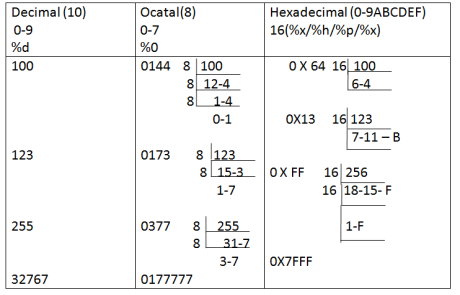10                                  012                                0X4 81X1X80X2 = 8X2   83                                  0 1 2 3                                              0X53 83X0+82X1+81X2+80X3 64+16+3       Error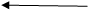0181 error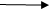Error 0981 range(0-7)   161                      0241                    0XA1 291                      0443                    0X123

Binary Number System :-

• The base value of binary Number system is 2 and the range 0,1
• When the memory will represented, always it holds in the form of binary.
• when we are representing the data in the binary complete data will be represented in the forms 1’s and ’0’s
• If there no any format specifiers are available to print binary representation on console

 Decimal Binary 1 0001 2 0010 3 0011 4 0100 5 0110 6 0110 7 1000 8 1000 9 1001
350010          0011 (32+2+1) 610011          1101 (32+16+8+4+1) 1270111          1111(64+32+16+8+4+2+1) 2551111           1111 327670111        1111          1111          1111 655351111          1111          1111          1111
 No.of bits rep No.of combinations max value max 1 2(21) 1(21-1) 01 2 4(22) 3(22-1) 11 3 8(23) 7(23-1) 0111 4 16(24) 15(24-1) 1111 5 32(25) 31(25-1) 0001 111 6 64(26) 63(26-1) 0011 1111 7 128(27) 127(27-1) 0111   1111 8 256(28) 255/-1(28-1) 1111 1111 9 65536(216) 65535/-1(216- 1111  1111 1111 1111 10

 8bits      -              1 Byte1024B    -              1KB 1024KB -               1MB 1024MB -             1GB 1024GB-               1TB

Int i:-

On Dos based compiler the size of int is 2 bytes and the range from  -32768 to + 32767 For representing any integer value, we require 16 bit combination i.e 65536 representations. Among those all combinations, 50% will represent negative data and remaining 50% will represents +ve data.
 8bits      -              1 Byte1024B    -              1KB 1024KB -               1MB 1024MB -             1GB 1024GB-               1TB

• Left side most signigicant bit is called signed bit is called signed bit
• Always signed bit will decides the return value of binary representation
• If sign bit is “0” and remaining b all bits are Zero’s then it gives minimum value of +ve sign ie ‘0’
• if sign bit is ‘0’ and remaining all bits are 1’s then it gives maximum value of +ve sign ie 32767
• If the sign bit is ‘1’ and remaining all bits are 0’s then it gives minimum value of –ve sign i.e 32768
• If the sign bit is ‘1’ and remaining all bits are 1’s then it gives maximum value of –ve sign ie -1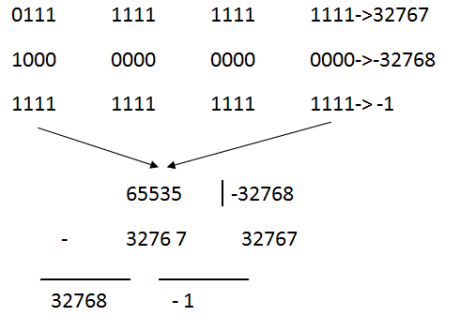int i; i=32767+1; 324670111     1111          1111          1111 10000      0000           0000           0001 1 1 1 1     1 1 1 1      1 1 1 1          1 1 10000         0 0 0 0         0 0 0         0 0 0

Unsigned integer of U:

The size of unsigned int is 4 bytes and the range from 0 to 65,535 For representing any unsigned integer value, we require 16 bit combination i.e 65536  representations.
• Among those all representations all gives +ve data only, because in unsigned type , signed bit is not available
• When we are working with integer and unsigned integer always format specifier will decide the return value of binary representation.
 %d Binary Representations %U 0 32767   -32768   -1 0000    0000   0000   0000 01111   1111   1111  1111   1000     0000   0000  0000   1111   1111    1111    1111 0 32767   32768   65535

Character of ch:-

• The size of characters is 1 byte and the range from -128 to +127
• For representing any character we require 8 bit combination i. e 256 representations.
• Among those all representations, 50% will gives +ve data and remaining 50% will give –ve data.
0000          00000 0111          1111127 1000          0000-128 1111          1111-1

Unsigned character of ch :-

The size of unsigned character is 1 byte and the range from 0 to 255   For representing any unsigned character we require 8 bit combination i.e 256 combinations and all the representation gives +ve data only.   0000        00000 0111          11111270000 1000          0000128 1111          1111255   255                 -128 (-)     127                 + 127 128                     -1     When we are working with char & unsigned char, always data type will decides the return value of binary representation.
 printf(“%d   %U”, 65535,-1); -1 65535 printf(“%d    %U”,32768,-32768); -32768 32768 printf(“%U   %O   %X ”,-1,-1,-1); 65535, printf(“%d   %U”, 32768,-32768); -32768 32768 printf(“%u   %O   %X”,-1,-1,-1); 65535 0177777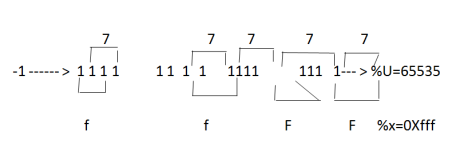For representing any octal data we require to take 3 binary bits only, for representing any hexadecimal value, we require to take 4 binary bits. printf(“%d  %0  %u”, 327684,327684,327684); o/p: -32768        0100000